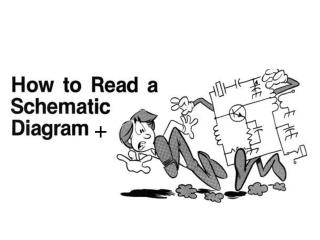DownloadDownload PresentationSimple Electrical Circuit pictorial

Simple Electrical Circuit pictorial

Télécharger la présentationSimple Electrical Circuit pictorial

- - - - - - - - - - - - - - - - - - - - - - - - - - - E N D - - - - - - - - - - - - - - - - - - - - - - - - - - -
Presentation Transcript

1. +

2. Simple Electrical Circuitpictorial

3. Simple Electrical Circuitschematic

4. A voltage divider used for volume control. Block Diagram Drawing

5. Standard symbol for a dc voltage source.

6. electrical schematic of flashlight

7. Resistance symbol and notation.

8. Film resistors:

9. FIG. 3.25 Color coding for fixed resistors.

10. Color-code bands on a resistor • 1st band is the first digit of the resistance value • 2nd band is the second digit of the resistance value • 3rd band is the multiplier (number of zeros) • 4th band indicates the tolerance

11. Color coding.

12. Standard Values of Resistors

13. Potentiometer control of voltage levels.

14. Potentiometer and rheostat symbols and basic construction of one type of potentiometer.

15. Typical potentiometers and two construction views.

16. Typical fuses and circuit breakers and their symbols.

17. Common Ground Symbol

18. Circuit Ground • Voltage is relative • The voltage at one point in a circuit is always measured relative to another point • This reference point in a circuit is usually the ground point

19. Notation Voltage sources and grounds Ground symbol Voltage source symbol

20. Symbols for ground.

21. A simple circuit with ground connections.

22. Using an ohmmeter to measure the total resistance of a series circuit.

23. Schematic representation for a dc series circuit.

24. Parallel combination of resistors.Same resistance value

25. Connections or NODES

26. Symbol for the inductor.

27. Typical Inductors

28. Relative sizes of different types of inductors: (a) toroid, high-current; (b) phenolic (resin or plastic core); (c) ferrite core.

29. Symbols for the capacitor: (a) fixed; (b) variable.

31. Capacitors Variable Capacitors • Most common are shown in the figure below. The dielectric for each is air. The capacitance is changed by turning the shaft at one end to vary the common area of the movable and fixed plates. The greater the common area the larger the capacitance.

32. Symbol for a sinusoidal voltage source. Volts A.C.

33. Schematic symbols specify the type of core.

34. Operational Amplifier

35. Op-amp symbols and packages.

36. European schematic design

37. European schematic design

38. Transistors

39. Amplifier Output stage

40. D.C. 5volt Power Supply

41. Connections Wire To pass current very easily from one part of a circuit to another A ‘DOT' should be drawn where wires are connected (joined), but it is sometimes omitted.

42. Connections • In complex diagrams it is often necessary to draw wires crossing even though they are not connected, the 'bridge' symbol shown on the right is preferred because the simple crossing on the left may be misread as a join where you have forgotten to add a ‘DOT'!